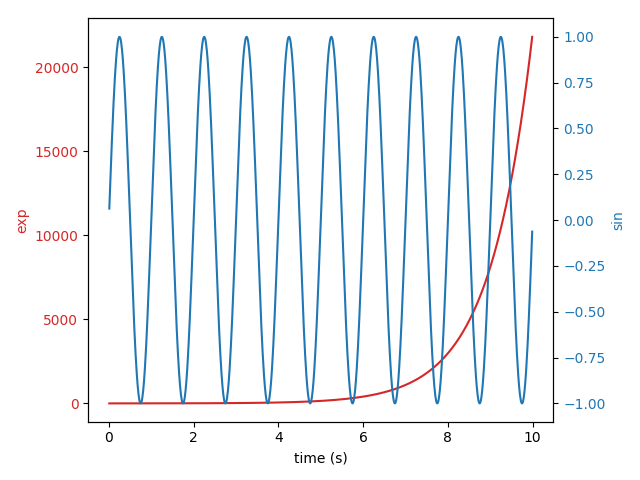# Plots with different scales¶

Two plots on the same axes with different left and right scales.

The trick is to use two different axes that share the same x axis. You can use separate `matplotlib.ticker` formatters and locators as desired since the two axes are independent.

Such axes are generated by calling the `Axes.twinx()` method. Likewise, `Axes.twiny()` is available to generate axes that share a y axis but have different top and bottom scales.

```import numpy as np
import matplotlib.pyplot as plt

# Create some mock data
t = np.arange(0.01, 10.0, 0.01)
data1 = np.exp(t)
data2 = np.sin(2 * np.pi * t)

fig, ax1 = plt.subplots()

color = 'tab:red'
ax1.set_xlabel('time (s)')
ax1.set_ylabel('exp', color=color)
ax1.plot(t, data1, color=color)
ax1.tick_params(axis='y', labelcolor=color)

ax2 = ax1.twinx()  # instantiate a second axes that shares the same x-axis

color = 'tab:blue'
ax2.set_ylabel('sin', color=color)  # we already handled the x-label with ax1
ax2.plot(t, data2, color=color)
ax2.tick_params(axis='y', labelcolor=color)

fig.tight_layout()  # otherwise the right y-label is slightly clipped
plt.show()
```